Laitinen, O., Kemppainen, K., Stoor, T., and Niinimäki, J. (2011). "Fractionation of pulp and paper particles selectively by size," BioRes. 6(1), 672-685.

#### Abstract

A study was made of the classification of pulp, paper, and peat particles by size with a device called a tube flow fractionator. An accurate and simple experimental model was formulated in order to estimate the time required for fractionation, yielding an excellent correlation between the observed and predicted fractionation times. The results showed that the fractionation time of a certain size of pulp, paper, and peat particles in the tube flow device can be accurately estimated from the length, width, and thickness of the particle. The results can be used to facilitate the selection of specific fractions of pulp and paper samples.

FRACTIONATION OF PULP AND PAPER PARTICLES SELECTIVELY BY SIZE

Ossi Laitinen,a,* Kalle Kemppainen,a Tuomas Stoor,a and Jouko Niinimäki a

A study was made of the classification of pulp, paper, and peat particles by size with a device called a tube flow fractionator. An accurate and simple experimental model was formulated in order to estimate the time required for fractionation, yielding an excellent correlation between the observed and predicted fractionation times. The results showed that the fractionation time of a certain size of pulp, paper, and peat particles in the tube flow device can be accurately estimated from the length, width, and thickness of the particle. The results can be used to facilitate the selection of specific fractions of pulp and paper samples.

Keywords: Tube flow fractionation; Modelling; MLR model; Fractionation time and pulp particles

Contact information: a: Fibre and Particle Engineering Laboratory, Department of Process and Environmental Engineering, P.O.Box 4300, FIN-90014 University of Oulu, Finland. *Corresponding author: ossi.laitinen@oulu.fi

INTRODUCTION

Fractional analysis of multicomponent samples such as pulps gives information about the properties of these samples and their particles that is difficult to obtain otherwise, e.g. the mass proportions of filler or pulp fractions, the attached and detached ink content of deinked pulp, and various particle properties accessible to image analysis (Laitinen et al. 2006, 2007a, 2008). One simple and fast but not very well known method of classifying paper pulp particles according to their dimensions is tube flow fractionation, where particle separation occurs by means of continuous water flow (Pascal and Silvy 1991; Silvy and Pascal 1992; Pascal and Silvy 1993; Krogerus et al. 2003).

In the tube flow technique the particles are separated axially so that the largest ones accumulate at the front end of the flow plug and the smallest ones at the rear end (Johansson and Kubat 1956; Mason 1950; Olgård 1970; Johansson et al. 1970). For efficient particle separation, the flow conditions should differ from a laminar flow profile. Reynolds numbers within the range 1000 to 10,000 for water are considered to be the most efficient for the separation of pulp particles (Pascal and Silvy 1993). The consistency of the sample must be below 0.5% (depending slightly on the pulp type), as no selective separation occurs above this level, since the fibres form a coherent network. The fractionation phenomenon used in tube flow fractionation differs from that in hydrodynamic chromatography (Provder 1997; Schimpf et al. 2000). In tube flow fractionation so-called lift forces play a more important role than they do in hydro-dynamic chromatography. This means in practice that particles try to keep away from the tube wall. Particles that settle against the bottom wall of the tube do not progress through the tube equally as the particles drifting within the water flow. The exact mechanism of particle separation under the non-laminar conditions of continuous water flow used in this research has not been verified, but some hypotheses can be advanced.

When the sample is injected into the tube, the particles are distributed randomly (see Fig. 1a). As the flow begins and proceeds, the slight turbulence in the transition flow regime begins to move the particles randomly inside the flow. It is assumed that small particles that occasionally drift close to the tube wall may not be captured easily by the faster middle flow and will thus spend a portion of the time in the zone where the flow velocity is lower. Large particles may also drift close to the tube wall but may be recaptured more easily by the faster middle flow due to their greater dimensions. The probability of being captured by the faster middle flow is higher for particles with one long dimension (e.g. fibres) or multiple long dimensions (e.g. paper flakes) than for particles with short dimensions (e.g. fines). Thus, the large particles tend to concentrate at the front end of the flow (Fig. 1b) and finally come out from the tube first (Fig. 1c).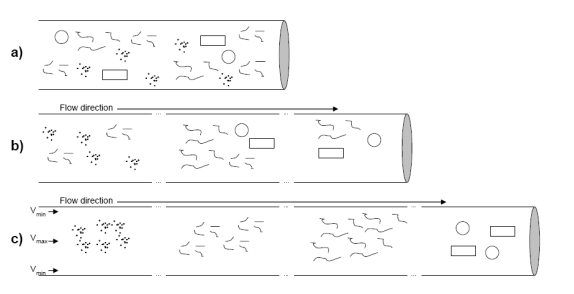Fig. 1. Schematic description of tube flow fractionation

The force that causes large particles in complex pulp suspensions to accumulate to the front end of the flow and small particles to the rear end is not fully understood; however, and it is very difficult to verify the effect of various shape factors from a theoretical point of view. The method has been observed to fractionate pulp particles mostly according to their length (Krogerus et al. 2003; Pascal and Silvy 1993), but fibre lengths do not exclusively explain the observed variation in fractionation time between pulp types (e.g. kraft pulp fibres versus mechanical pulp fibres manufactured from identical raw materials). Thus, it can be assumed that other particle dimensions and surface properties also affect particle separation in a tube flow environment, although the significance of these effects is still unclear. Additionally, there are no simple models available that accurately estimate the time needed for a particle to be carried along the tube.

The aim of this research was to study the effect of particle dimensions (length, width, and thickness) on separation in tube flow. The target was to estimate the time at which a particular particle would come out of the tube based on knowledge of its dimensions and to build a simple experimental model to describe that time, i.e. the fractionation time. The validity of the model was verified with a number of pulp, paper, and rectangular peat samples. The results indicate that the fractionation time of a particle in tube flow can be estimated accurately from its particle dimensions.

MATERIALS AND METHODS

Materials

The materials used were various pulp, paper, and peat particles (see Table 1), dimensional analyses of which are presented later in this section. Peat is harvested as a typical source of fuel in Finland. The pulp samples were obtained from various manufacturing processes: Pine softwood kraft, eucalyptus hardwood kraft, thermo-mechanical pulp (TMP), groundwood pulp (GW), and deinked pulp (DIP). In addition, pieces of copy paper of different dimensions were also investigated. The results are based on 181 independent sample points (of which 69 were used to create the empirical model and 112 for subsequent verification).

Table 1. Particles Used and their Dimension Ranges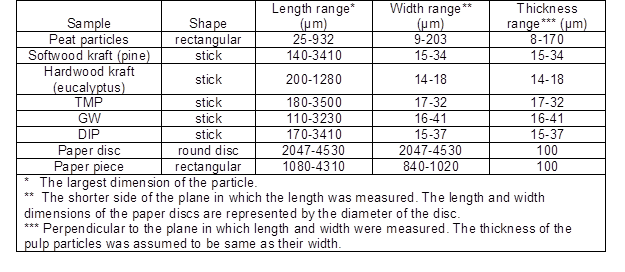Since the peat samples included very large particles, a decision was made to grind the samples in order to obtain smaller sizes (mostly under 1000 µm). After pre-treatment, the ground peat samples were then divided into size categories by means of sieve shakers (Retsch) of mesh 32 µm, 45 µm, 63 µm, 90 µm, 125 µm, 250 µm, and 500 µm in order to achieve narrow particle size distributions.

Copy paper (grammage 80 g/m²) was used in order to obtain samples with a uniform shape. This was achieved by cutting rectangular pieces using a paper guillotine and small, round discs of various size categories (diameter from 2 mm to 4.5 mm) using perforator blades.

The pulp samples were fractionated into size categories by the tube flow fractionation method, which is known to fractionate all pulp samples selectively, from the largest fibres to the finest particles. The tube flow fractionator also allowed small intermediate fibre fraction samples to be taken after fractionation with a very narrow length distribution.

Particle Dimension Analysis

The fractionated pulp fibres were measured with a FiberLab (Metso Automation) fibre analysing device in order to determine basic properties such as length, width, and thickness (TAPPI T271 2002; Tiikkaja 2007). The FiberLab measures individual fibres with two cameras at 10 μm and 1.5 μm resolutions and reports the length results according to either the TAPPI (T271) or the ISO standard (ISO-16065). Since it is not possible to measure the thickness of particles (perpendicular to the field of view) from images, the thicknesses of the fibres were assumed to be same as their widths.

Paper samples and peat particles were studied under a microscope (Leica DFC320) and photographed with a digital camera. Each sample was photographed using a fixed picture ratio in which the transformation ratio from pixel to µm was determined to ensure that all data were expressed on a metric scale. The images were then measured with the Matrox Inspector image analysis software to determine the length and width of the particles.

In addition, a certain number of pieces of paper and peat particles were placed between glass slides in order to determine their thickness using a laboratory thickness meter (Lorenzen & Wettre).

Equipment Used

Tube flow fractionation

The tube flow fractionator used in the experiments (Laitinen et al. 2006, 2007b) employs a method in which a defined amount of pulp or sample (volume of 50 mL with a consistency of 0.3%, which is the equivalent of 150 mg absolute dry sample) is injected into the water flow through a 100 meters long plastic tube wound onto a wheel. When the pulp-water mixture exits the long tube it is divided into size fractions according to the setup of the device. The fibre fractions can then be analysed with other equipment such as a fibre analyser or microscope. A schematic view of the fractionation apparatus and measurement devices is provided in Fig. 2.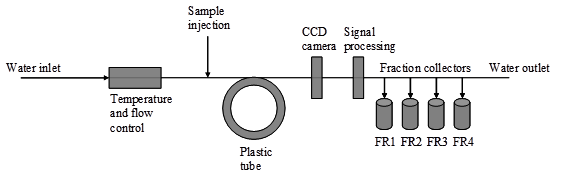Fig. 2. Schematic view of the tube flow fractionator

The variables of interest during fractionation, including flow velocity, pressure, temperature, sample volume, and consistency, were all maintained precisely at a constant level, as each variable has a direct effect on the fractionation. The essential variables and parameters during fractionation are presented in Table 2. Of these variables, flow velocity, pressure, and temperature were defined by the manufacturer of the apparatus, Metso Automation.

Table 2. Parameters and Variables of Tube Flow Fractionation Device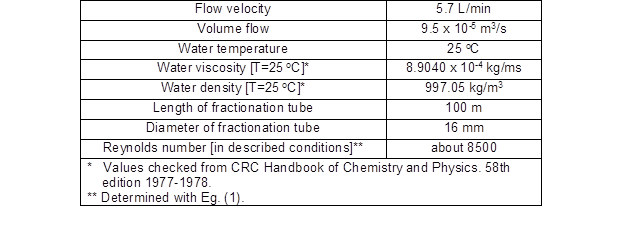It is well known that when the flow velocity and temperature are both constant, certain fibre sizes always exit the flow tube at the same time. Besides the fractionation time, it is also possible to regard the amount of water that has flowed through the fractionation hose as a relevant parameter. Thus the fractionation results can be expressed as a function of either fractionation time or the volume of water that has flowed through the tube.

The particles were fractionated at a flow rate of 5.7 L/min with a Reynolds number of around 8500 (Liukkonen 2006). This flow rate enables all of the particles to be separated by size during the fractionation process. The Reynolds number can be determined using Eq. (1),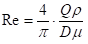(1)

where Q is the volume flow and ρ is the density of fluid (water). In addition, D is the diameter of the fractionation tube and μ is the dynamic fluid viscosity (water).

Typical results

A typical elution curve of a TMP sample is presented in Fig. 3. Below the elution curve, examples of TMP particles are presented after certain fractionation times (175 s, 185 s, and 195 s). After fractionation in the tube, the optical consistency transmitter and the CCD camera are activated (see Fig. 2). About 3000 depolarization and absorption signal values are collected while the sample flows through the optical consistency transmitter. After the consistency measurement point, about 600 images are also recorded as the pulp sample passes the camera (Laitinen et al. 2006).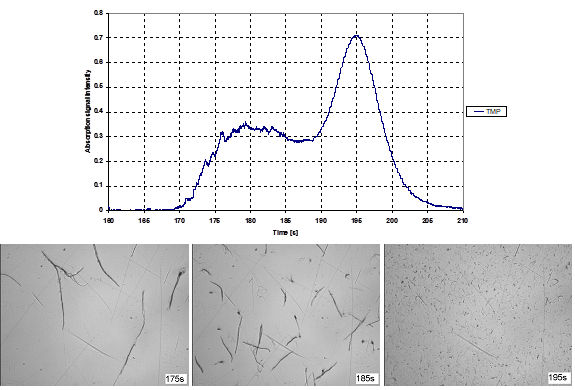Fig. 3. Typical elution curve of TMP sample and examples of TMP particles after certain fractionation times (175 s, 185 s, and 195 s).

Data Analysis and Modelling

MODDE 7.0 (Umetrics), a program developed for experiment design and data analysis, was used to analyse the data on the fractionation, FiberLab measurements, and microscopic analysis. A multilinear regression (MLR) model was fitted to the experimental data and the best model was identified. The quality of the model was then evaluated from a statistical point of view.

RESULTS

Master Model and Statistical Analysis

The subset data series (creating the model and the verification period of the final model) contained particles from as wide a particle size range as possible in order to obtain a practical model. Referring back to Table 1, it can be seen that the experiments were conducted on a variety of pulp, paper, and peat particles on which FiberLab measurements (of pulp fibre dimensions) and microscopic analyses (of peat particles and pieces of paper) had been performed. Based on these observations, a master model was created to estimate fractionation times for particles with different lengths, widths, and thicknesses in tube flow fractionation. The master MLR model for tube flow fractionation time, determined by MODDE, is presented in Eq. (2).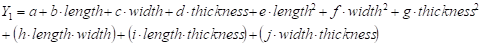(2)

The scaled coefficients and P-values of the master model are presented in Table 3. As can be seen, all the P-values were under the selected critical P-value (0.05), which means that all factors would be statistically significant factors in the model. Even if all the interaction terms and second order terms were statistically significant, most of them would not have any practical influence on the fractionation time in a tube flow fractionator. Thus, the complex master model could be simplified while still obtaining an equally good result as with the presented master model.

Table 3. Scaled Coefficients and P-values of the Master MLR model. Certain key factors of the master MLR Model are included in the lowest part of table.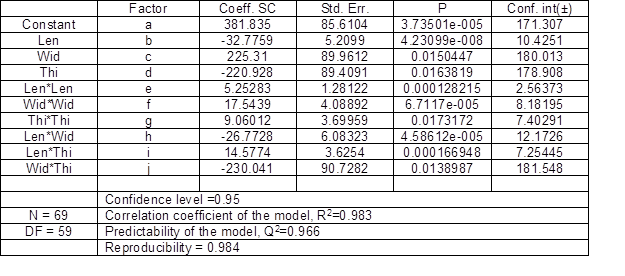Simplified MLR Model and Statistical Analysis

As mentioned in the previous section, the master model could be simplified by dropping most of the interaction terms and second order terms. The simplified MLR model is shown as Eq. (3) and the essential factor coefficients of the simplified model in Table 4.(3)

Table 4. Coefficients of the simplified MLR modelThe significance of each simplified MLR model parameter is presented in Fig. 4. The coefficient plot displays the regression MLR coefficients with their confidence intervals. It can be seen that all selected parameters are significant (different from the noise), because the confidence interval does not cross zero.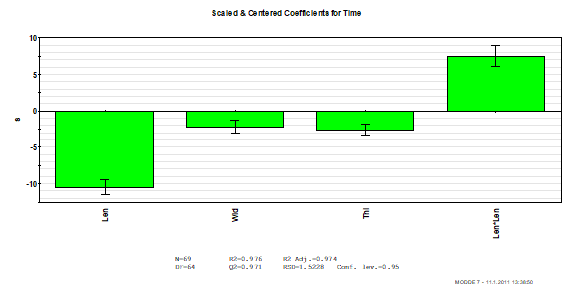Fig. 4. Significance of each simplified MLR model parameter

Since the correlation between the predicted and observed fractionation times, as presented in Fig. 5, is linear, the MLR model (created using 69 sample points representing pulp, paper, and peat particles of different sizes) seems to be in good agreement with the measurements and can be used to predict the fractionation times of various pulp, paper and peat particles.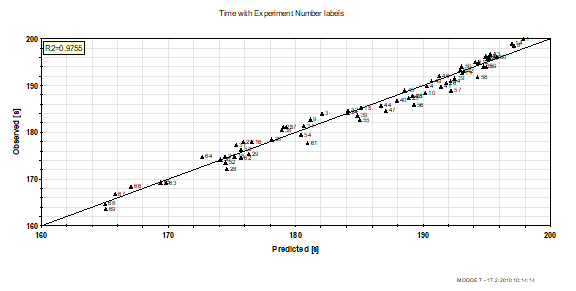Fig. 5. Correlation between predicted and observed fractionation times

A normal probability plot for the calculated residuals (differences between the observed and predicted fractionation times) is presented in Fig. 6. This figure indicates whether the data include outliers that point to defects in the model. Since the residuals form a straight line between -4 and +4 on the x-axis (studentized deviation) in the normal probability plot, it can be concluded that there were no outliers and the model fits well with the measured data.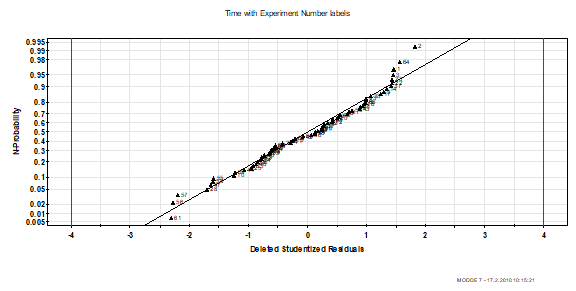Fig. 6. Normal probability plot of the measured residuals

The basic characteristics R², Q², and reproducibility of the simplified MLR model are presented in Table 5. R² is a measure of fit, i.e. how well the model fits the measured data, whereas Q² indicates how well the model predicts new data points. A good model should have both a high R² and a high Q². Finally, reproducibility is the variation in the response under the same conditions (pure error), as compared with the total variation in the response. It can be seen that the key factors in the simplified MLR model are at the same level as those in the complex master model (compare Table 3).

Table 5. Certain Key Factors of the Simplified MLR ModelThe effects of different particle dimensions in the studied range of dimensions (see Table 1) on the fractionation time (middlemost) are presented in Fig. 7. The graphs are plotted so that one dimension is plotted at a time and the other two dimensions are kept at the average values for the investigated data (length=1700µm, width=70µm, and thickness=40µm). The graphs clearly show that the fractionation time decreases as the particle dimensions increase. It was particle length, however, that had the most significant effect on fractionation time in the tube flow (see also Fig. 4). In addition, Fig. 7 includes plots of the lower and upper confidence limits (at the 95% confidence level) of the predicted effects of the various particle dimensions on a given factor.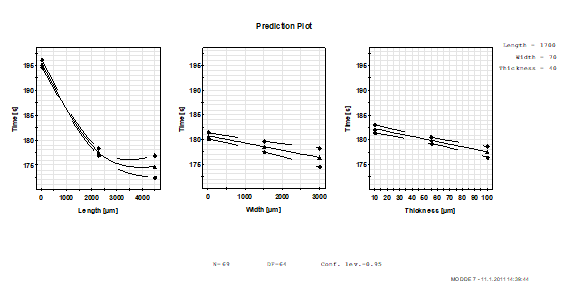Fig. 7. Prediction plots of the dimension variables (length, width and thickness)

Verification of the Final MLR Model

After formulation of the final MLR model for fractionation time in a tube flow fractionator (see Eq. 3), this model was used in the subsequent verification phase (with 112 independent sample points not used in the modelling phase) to investigate the accuracy of the model in predicting new data points. The correlation between the predicted and observed fractionation times is shown in Fig. 8 for the various pulp size fractions, pieces of paper (i.e. round discs and rectangular pieces) and peat samples (sieved into size categories). As can be seen, the model successfully predicted the fractionation times of the pulp, paper, and peat samples of various sizes. In general, the fractionation time decreased as the particle dimensions increased.

DISCUSSION

Although the tube flow fractionation method has been found to fractionate pulp particles primarily according to their hydrodynamic length (Krogerus et al. 2003; Pascal and Silvy 1993), there still has been a lack of systematic knowledge regarding the effect of the other particle dimensions (i.e. width and thickness) on the fractionation time. Thus more detailed information is needed on these. According to previous studies, changes in fibre length do not exclusively explain the variation in fractionation time observed between pulp types (e.g. in kraft pulp fibres versus mechanical pulp fibres). This study focused on clarifying the contribution of the various pulp, paper and peat particle dimensions to the fractionation time by means of simple empirical modelling.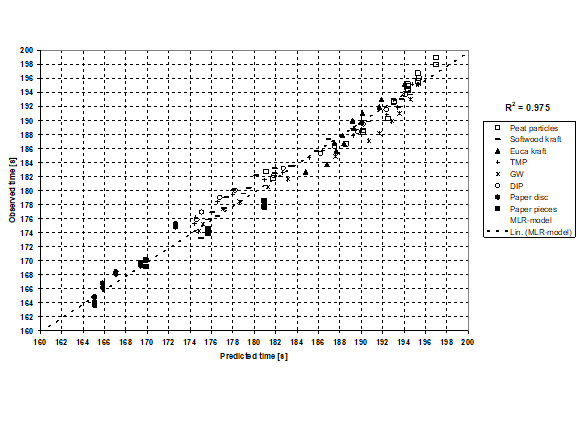Fig. 8. Correlation between predicted and observed fractionation times during the verification period

Simplified MLR Model and Statistical Analysis

The multilinear regression (MLR) model was developed to estimate the fractionation time. All the selected dimensions (length, width, and thickness) can be determined independently from each other. The exceptions were image analyses, where the thickness was assumed to be the same as the width. The newly developed model for the predicted fractionation time gave a linear correlation with the observed fractionation time (see Fig. 5).

It can be seen from Fig. 6 that all the measured data points lie either on or very close to a straight line between -4 and +4 along the x-axis (studentized deviation). This means in practice that the residuals were almost completely evenly distributed and no systematic error existed in the data.

The characteristics of the simplified MLR model (R², Q² and reproducibility) are presented in Table 5. R² is the percentage of the variation in the response that is explained by the model, which describes how well the model fits with the data. Q² is the percentage of variation in the predictions given by the model according to cross-validation, and indicates how well the model will predict new data, while reproducibility is the variation in the response under the same conditions (pure error), as compared with the total variation in the response. All the characteristic values were very high (>0.97), which indicated that the model was valid for this fractionation analysis.

The developed model can be used to estimate the effect of pulp, paper, and peat particle dimensions on the fractionation time (see Fig. 7). It was shown that particle length had the most significant effect on fractionation time in a tube flow environment within the range of dimensions examined here (see Table 1, Fig. 4 and Fig. 7), but the data also suggested that the plot of particle length versus time is not linear over the entire length range (Fig. 7). It should be noted that in practice there were no pulp fibres in the over 3500 µm category. All the particles in this size category were pieces of paper pieces, and their shapes were far from elongated (like the fibres). Furthermore, the statistical confidence limits for these larger particles were poorer than for the smaller particles on account of the smaller number of data points. Therefore, for fibre-like particles in their longest dimension over 3500 µm, further studies would be required to verify the curvature in the effect of length. In addition to particle length, the width and thickness of a particle clearly affected the fractionation time (see Figs. 4 and 7). Overall, the fractionation time became shorter as the mean dimensions of the particles increased.

It can be assumed that the slight turbulence in the transient flow conditions in this fractionation system moves the particles randomly inside the flow. Some particles drift close to the tube walls, whereas others stay in the middle of the tube. Of the particles that are close to the tube wall, the larger ones have a higher probability of being captured by the faster moving middle flow than the smaller ones, due to their larger dimensions. The more large dimensions a particle has, the higher is the probability of its being captured by the faster moving middle flow, since the orientation of particles in the flow field is not such a significant factor for these particles than it is for fibre-like particles. One other explanation would be that the large particles rarely drift close to the tube walls and thus are rarely located in the lower-velocity flow. These hypotheses could explain why large, flake-like particles (in this case the paper discs and paper pieces) come out of the tube faster than fibre-like particles, while fibre-like particles come out faster than fines.

Verification of the Final MLR Model

The MLR model was verified with pulp, paper, and peat particles of different types and representing a wide size range (see Fig. 8), and proved to be valid for all the particles that typically kept away from the tube wall. It should be noted that the model is not valid for large particles of a density that is much greater than that of water, since these particles are likely to settle against the bottom wall of the tube and be unable to drift within the water flow. Therefore special attention should be paid to the range of dimensions (see Table 1), since the model is not necessarily valid outside of this range.

It is generally known that each pulp particle size category has a different impact on the final pulp properties. The model formulated here can help one to calculate the specific pulp, paper and peat particle fractions in a sample beforehand, given that the essential dimensions of the particles (length, width and thickness) are somehow known. Thus it can be of help in situations where the number of flake-like pieces in a pulp sample must be known, for example, since these will leave the tube faster than smaller individual long fibres.

CONCLUSIONS

This study was undertaken in order to investigate the effects of pulp, paper, and peat particle dimensions on fractionation time during tube flow fractionation. A Multilinear Regression (MLR) model was developed to estimate the fractionation times of pulp, paper, and peat particles of various dimensions by means of particle length, width and thickness. Overall, it is particle length that has the most significant effect on the fractionation time, while particle width and thickness have a smaller, but still statistically significant effect (at the 95% confidence level). The validity of the model was very good and it can be used to predict new sample points accurately and to facilitate the selection of a fractionation setup for further specific analyses of given pulp types.

REFERENCES CITED

Johansson, B., and Kubat, J. (1956). “An axial separation effect in flowing pulp suspensions,” Svensk Papperstidn. 59(23), 845-846.

Johansson, H., Olgård, G., and Jernqvist, Å. (1970). “Radial particle migration in plug flow − A method for solid-liquid separation and fractionation,” Chem. Eng. Sci. 25(3), 365-372.

Krogerus, B., Fagerholm, K., and Löytynoja, L. (2003). “Analytical fractionation of pulps by tube flow,” Pap. Puu-Pap. Timber 85(4), 209-213.

Laitinen, O., Löytynoja, L., and Niinimäki, J. (2006). “Tube flow fractionator − A simple method for laboratory fractionation,” Pap. Puu-Pap. Timber 88(6), 351-355.

Laitinen, O., Löytynoja, L., Kumpulainen, H., and Niinimäki, J. (2007a). “Method and measuring device for measuring recycled fibre pulp,” International Patent, Publication number: WO 2007/122289.

Laitinen, O., Körkkö, M., Ämmälä, A., Saren, M., and Niinimäki, J. (2007b). “Analysis of pulp component in a DIP process with tube flow fractionation”, Proc. 8th Research Forum on Recycling, Tappi 2007, Niagara Falls, Canada, 23-26 September 2007, 272-281.

Laitinen, O., Körkkö, M., and Niinimäki, J. (2008). “The effects of aging in different raw material furnishes,” Prog Pap Recycling 18(1), 9-15.

Liukkonen, J. (2006) “Determination of paper furnish flocculation with a fractionator,” MSc thesis, Chemical Technology, Lappeenranta University of Technology (in Finnish).

Mason, S. G. (1950). “The motion of fibres in flowing liquids,” Pulp Pap.-Can, April 1950, 93-100.

Olgård, G. (1970) “Fractionation of fiber suspensions by liquid column flow,” Tappi J, 53(7), 1240-1246.

Pascal, R., and Silvy, J. (1991). “La caractérisation en continu des pates à papier,” Revue A.T.I.P. 45(7), décembre 1991, 253-261 (in French).

Pascal, R., and Silvy, J. (1993). “Hydrodynamic fractionation applied to characterization of paper pulp,” Récents progres en génie des procédés 7(29), 233-238 (in French).

Provder, T. (1997) “Challenges in particle size distribution measurement past, present and for the 21st century” Prog. Organic Coatings 32(1997), 143-153.

Schimpf, M., Caldwell, K., and Giddings, J. (2000) “Field flow fractionation handbook,” A Wiley-Interscience publication, USA 2000, 592 p.

Silvy, J. and Pascal, R. (1992). “Device for determining the characteristics of particles in suspension in a liquid,” U.S. Patent, Publication number: 5,087,823.

TAPPI T271 om-02 “Fiber length of pulp and paper by automated optical analyzer using polarized light 2002.”

Tiikkaja, E. (2007) “Application of an optical fibre analyser and a tube flow fractionator to the estimation of quality potential of TMP – Experimental study,” Acta Univ. Oulu C, 285 (in Finnish).

Article submitted: October 25, 2010; Peer review completed: December 13, 2010; Revised version received and accepted: January 13, 2011; Published: January 15, 2011.